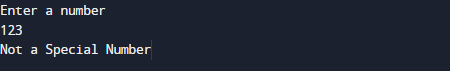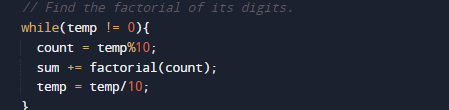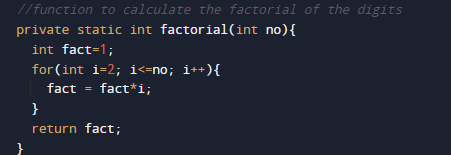# Program to check Special Number Program in Java

In this tutorial you will learn about the Program to check Special Number Program in Java and its application with practical example.

In this tutorial, we will learn to create a Java Program to check Special Number Program in Java

## Prerequisites

Before starting with this tutorial we assume that you are best aware of the following Java programming topics:

• Java Operators.
• Basic Input and Output function in Java.
• Class and Object in Java.
• Basic Java programming.
• If-else statements in Java.
• loops in java

# Special Number in Java

Number said to special if the sum of  factorial of its digits is equal to the Given number.

Example: 145.
-> factor of 1! + factor of 4! + factor of  5!
-> 1 + 24 + 120.
-> 145.

So the number is a special number.

## Program to check Special Number Program in Java

In this program we would find the given number is special number or not , first will take the input from the user and then we calculate  sum of factor of number gives number it self it is Special number. Let have a look at the code.

## Output

Special Number.Not a Special Number.In the above program, we have first declared and initialized a set variables required in the program.

• number = it will hold entered number.
• temp = it  will hold temp the value of number.
• sum =  for adding values
• count= count will count size of a number
• i= For iteration.

After Declaring values first we will take a number form userand  pass number to temp variable where we break the numberand find factor of its each digit and calculate sum of all digit.if sum of all digit is equal to the original number the given number is a Special NumberAs shown in image if number equal to the sum of digit factor then it is  Special if not not a Special number.

In this tutorial we have learn about the Program to check Special Number Program in Java and its application with practical example. I hope you will like this tutorial.